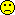## Who can help? [R for BE/BA]

Important update, I forgot vBR on the diagonal. Now the singuality is less of a heacahe:

 rm(list=ls(all=TRUE)) ###### par vector is c(varT, varBR, varWR) ###### ######   Section 1: Household things ###### CreateX=function(D) {  ##first the two treatments  TrtT=as.numeric(as.character(D$Trt)=="T") TrtR=as.numeric(as.character(D$Trt)=="R")  X=cbind(TrtT, TrtR)  R= qr(X)$rank ## subjects: in a mixed model with subj as random ## we do not do subjects also as fixed, therefore they are #'ed away here ## for (s in unique(D$Subj))  ## {  ## v=as.numeric(D$Subj==s) ## #print(v) ## XX=data.frame(X, v) ## names(XX)[ncol(XX)] = paste("S", s, sep="") ## rnk=qr(XX)$rank  ## if (rnk>R)   ##  {   ##     X=XX   ##     R=rnk   ##  }   ## }  ##now the Pers  for (p in unique(D$Per)) { v=as.numeric(D$Per==p)   #print(v)   XX=data.frame(X, v)   names(XX)[ncol(XX)] = paste("P", p, sep="")   rnk=qr(XX)$rank if (rnk>R) { X=XX R=rnk } } for (q in unique(D$Seq))  {   v=as.numeric(D$Seq==q) #print(v) XX=data.frame(X, v) names(XX)[ncol(XX)] = paste("Q", q, sep="") rnk=qr(XX)$rank   if (rnk>R)     {        X=XX        R=rnk     }  }  return(as.matrix(X)) } Create.CovM=function(Params) ##block diagonal covariance matrix {   varT=Params   varBR=Params   varWR=Params   #varRT=Params   #cat("Vars:", varT, varBR, varWR,"\n")   Nobs=length(D$Y) V=matrix(0,ncol=Nobs, nrow=Nobs) for (iRow in 1:Nobs) for (iCol in 1:Nobs) { ## the diagonal if (iCol==iRow) { if (D$Trt[iRow]=="T") V[iRow,iCol]=V[iRow,iCol]+varT       if (D$Trt[iRow]=="R") V[iRow,iCol]=V[iRow,iCol]+varWR +varBR } ## off diagonal if (iCol!=iRow) if (D$Subj[iRow]==D$Subj[iCol]) { if (D$Trt[iCol]==D$Trt[iRow]) V[iRow,iCol]= V[iRow,iCol]+varBR #if (D$Trt[iCol]!=D$Trt[iRow]) V[iRow,iCol]= V[iRow,iCol]+varRT } } return(as.matrix(V)) } ###### ###### Section 2: Matrix things ###### Obj.F12=function(Pars) ##this is line 3 of page 10 of: ##http://people.csail.mit.edu/xiuming/docs/tutorials/reml.pdf { CovM=Create.CovM(Pars) A= -0.5*log(det(CovM)) B= -0.5*log(det(t(X) %*% solve(CovM) %*% X)) est.b = solve(t(X) %*% solve(CovM) %*% X) %*% t(X) %*% solve(CovM) %*% y tmp= y - X %*% est.b C=-0.5 *(t(tmp) %*% solve(CovM) %*% tmp) return(A+B+C) } Some.Initial.Guesses=function(foo) { #guess variance within R D1=subset(D, D$Trt=="R")   m=lm(log(Y)~factor(Subj)+factor(Seq)+factor(Per) , data=D1)   #print(anova(m))   varWR= anova(m)["Residuals", "Mean Sq"]   m=lm(log(Y)~factor(Subj)+factor(Seq)+factor(Per)+factor(Trt) , data=D)   varBR= anova(m)["factor(Subj)", "Mean Sq"]   ##total var T, cheaply   ##actually, this may be much better: varT=varBR+varWR   D2=subset(D, D$Trt=="T") varT=var(log(D2$Y))   varT=varBR+varWR   L=list(varT=varT, varBR=varBR, varWR=varWR)   return(L) } #### #### section 3: execution #### D=read.csv("EMAII.a.csv" , header=T, sep="\t") ##public = easier X=CreateX(D) ##public = easier y=log(D$Y) ##public = easier Check.Surf=function(i, x1,x2, N) ## Checks the log likelihood surface of the i'th variance component ## (given the other variance components) for the interval var = x1 to x2 ## with a granularity of N points. { L=Some.Initial.Guesses(0) parIni=c(L$varT, L$varBR, L$varWR )   x=x1;   vX=NULL   vY=NULL   dx=(x2-x1)/N   for (j in 1:N)   {     vX=c(vX, x)     parIni[i]=x     vY=c(vY, Obj.F12(parIni))     x=x+dx   }   plot(vX, vY) } MyREML=function(foo.bar) ## ideally this would work, but it doesn't right now## also, I have a feeling the surface may be quite rugged, ## so I may switch to a homecooked optimiser ## once I have ironed out the singularity issue, see below. {   L=Some.Initial.Guesses(0)   print(L)   parIni=c(L$varT, L$varBR, L\$varWR)   F=optim(par=parIni, fn=Obj.F12, #method="L-BFGS-B",lower=c(0,0,0),       control=list(reltol=1e-10, trace=T))   print(F) } # if ti worked it'd be called through  MyREML(0) # however: Check.Surf(3, 0.01, 0.2, 50) ##checks the surface in one dimension # # # 

I could be wrong, but...

Best regards,
ElMaestro

No, of course you do not need to audit your CRO if it was inspected in 1968 by the agency of Crabongostan.Ing. Helmut Schütz